01/13 12:03

# 太长不看版

Predicting Clicks: Estimating the Click-Through Rate for New Ads

• CTR相关特征：
• Term CTR：包含相同关键词的其他广告的CTR
• Related Term CTR：包含相近关键词的其他广告的CTR
• 新广告本身特征
• Appeareanc：广告本身是否美观
• Attention Capture：广告是否有吸引力
• Reputation：广告主的知名度
• Landing Page Quality：登陆页的质量（我理解为广告引流页的质量）
• Relevance：广告和用户检索词 (search query)的相关性
• 广告指向的明确性：
• 作者认为指向越明确的广告CTR越高
• 外部特征：
• 关键词在网络上的词频
• 关键词在搜索引擎上的词频

# Motivation

## 为什么要预测广告CTR?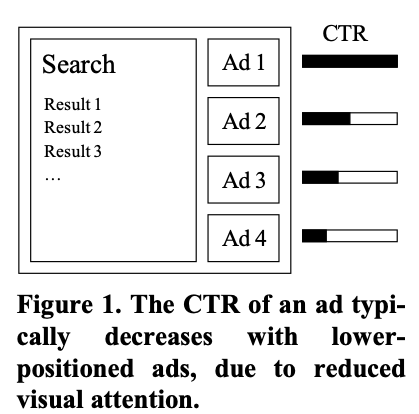# 广告搜索框架

• 用户看到它的可能性：由广告出现的位置(pos)决定
• 用户看到它之后点击它的可能性：由它本身质量决定

p ( c l i c k ∣ a d , p o s ) = p ( c l i c k ∣ a d , p o s , s e e n ) ∗ p ( s e e n ∣ a d , p o s ) p(click|ad, pos) = p(click|ad,pos,seen)*p(seen|ad,pos) p(clickad,pos)=p(clickad,pos,seen)p(seenad,pos)

p ( c l i c k ∣ a d , p o s ) = p ( c l i c k ∣ a d , s e e n ) ∗ p ( s e e n ∣ p o s ) p(click|ad, pos) = p(click|ad,seen)*p(seen|pos) p(clickad,pos)=p(clickad,seen)p(seenpos)

# 作者提出来的新特征

## 1. Estimating Term CTR

1.1 Term CTR

f 0 ( a d ) = α C T R ‾ + N ( a d t e r m ) ∗ C T R ( a d t e r m ) α + N ( a d t e r m ) f_{0}(ad) = \frac{\alpha \overline{CTR} + N(ad_{term} )*CTR(ad_{term})}{\alpha +N(ad_{term} )} f0(ad)=α+N(adterm)αCTR+N(adterm)CTR(adterm)

• C T R ‾ \overline{CTR} CTR 是训练集中所有广告的CTR的平均值(先验特征)
• α \alpha α 是先验特征的比例
这两项的作用是针对新广告中有未出现过关键词或者低频关键词。
• N ( a d t e r m ) N(ad_{term}) N(adterm) 是包含相同关键词的其他广告的数量(忽略词序)，
• C T R ( a d t e r m ) CTR(ad_{term}) CTR(adterm) 是包含相同关键词的其他广告的平均CTR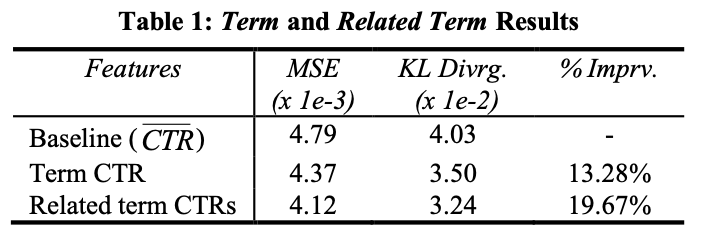1.2. Related Term CTR

R m n ( t ) = { ∣ a d i t e m ∩ t ∣ > 0    a n d ∣ t − a d i t e m ∣ = m    a n d ∣ a d i t e m − t ∣ = n R_{mn}(t) = \left\{ \begin{array}{lr} |ad_{item} \cap t| > 0 \space \space and& \\ |t - ad_{item} | = m \space \space and\\ |ad_{item} - t| = n & \end{array} \right. Rmn(t)=aditemt>0  andtaditem=m  andaditemt=n

C T R m n ( t e r m ) = 1 ∣ R m n ( t e r m ) ∣ ∑ x ∈ R m n ( t e r m ) C T R x CTR_{mn}(term) = \frac{1}{|R_{mn(term)}|} \sum_{x\in R_{mn(term)}} CTR_{x} CTRmn(term)=Rmn(term)1xRmn(term)CTRx

v m n ( t e r m ) = ｜ R m n ｜ v_{mn}(term) = ｜R_{mn}｜ vmn(term)=Rmn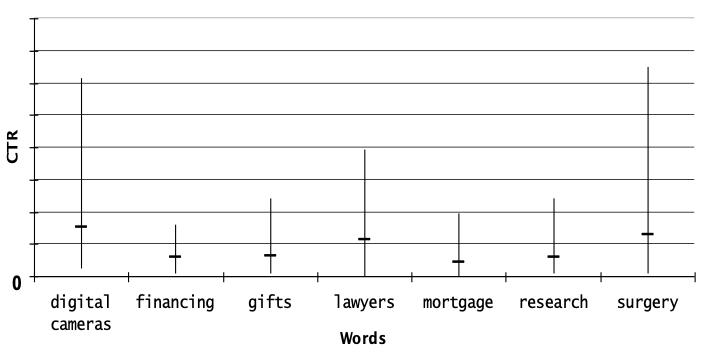2.1. Appearance

2.2. Attention Capture

2.3. Reputation

2.4. Landing page quality

2.5. Relevance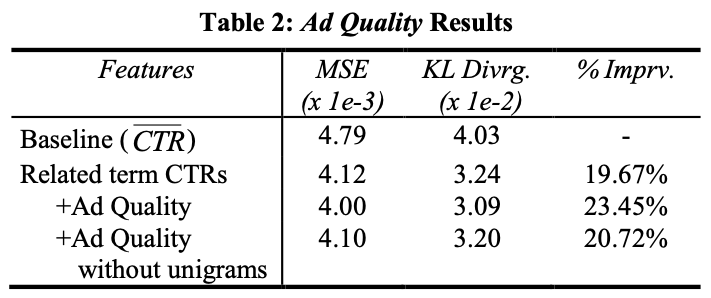## 3. Measuring Order Specificity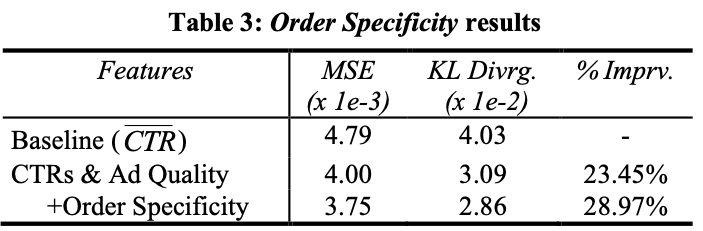## 4. External Sources Of Data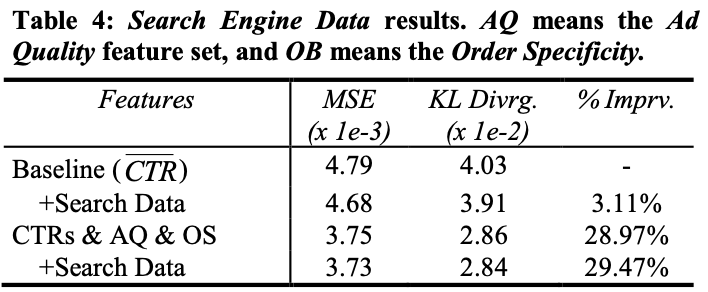# 数据集

• Landing page：登陆页
• Bid term：关键词
• Title：广告的标题
• Body：广告的内容
• Display URL：广告的超链接
• Clicks：自投放以来广告被点击的数量
• Views：自投放以来广告被看到的数量

# 模型

C T R = 1 1 + e − Z ,   Z = ∑ i w i f i ( a d ) CTR = \frac{1}{1+e^{-Z}} ,\space Z = \sum_{i}{w_i f_i(ad)} CTR=1+eZ1, Z=iwifi(ad)

• 增加了一个衍生特征 l o g ( f 1 + 1 ) log({f_1+1}) log(f1+1) f 1 2 {f_1}^2 f12：目的是防止有些特征最小值为0。
• 特征数据做标准化：目的是防止离群数据的影响(使用的是训练集上的均值和方差)
• 超过标准差5倍的数据做截断：超出5倍的数据按照5倍来计算，防止特征数据的长尾效应

# 自己的一点思考

• 构建新广告的特征考虑较为全面，不仅考虑了广告本身的吸引力，而且考虑了一些外部条件对广告的影响。
• 分析实验很“控制变量”，对于每一类新特征作者都用单独的实验验证其了有效性，并且分析了他们之间可能存在的overlap(覆盖)的情况
• 现在信息流推荐大家其实比的就是特征工程，谁的特征做得好，效果往往就会更显著。在本文中作者发现，其实一些统计特征的效果比手工特征来的更有效。手工特征往往加入了太多工程师自身对业务的理解，而统计数据才是最贴近用户行为的。

0
0 收藏

### 作者的其它热门文章0 评论
0 收藏
0